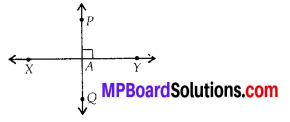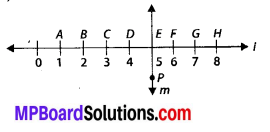# MP Board Class 6th Maths Solutions Chapter 5 Understanding Elementary Shapes Ex 5.5

## MP Board Class 6th Maths Solutions Chapter 5 Understanding Elementary Shapes Ex 5.5

Question 1.
Which of the following are models for perpendicular lines:
(a) The adjacent edges of a table top.
(b) The lines of a railway track.
(c) The line segments forming the letter’L’.
(d) The letter V.
Solution:
(a) Perpendicular
(b) Not perpendicular
(c) Perpendicular
(d) Not perpendicularQuestion 2.
Let $$\overline{P Q}$$ be the perpendicular to the line segment $$\overline{X Y}$$. Let $$\overline{P Q}$$ and $$\overline{X Y}$$ intersect in the point A. What is the measure of ∠PAY?
Solution:
∠PAY = 90°Question 3.
There are two set-squares in your box. What are the measures of the angles that are formed at their corners? Do they have any angle measure that is common?
Solution:
One set-square has angles 45°, 90°, 45° and other set-square has angles 60°, 90°, 30°.
Yes, they have angle measure 90° as common.Question 4.
Study the diagram. The line l is perpendicular to line m
(a) Is CE = EG?(b) Does PE bisect CG?
(c) Identify any two line segments for which PE is the perpendicular bisector.
(d) Are these true?
(i) AC>FG
(ii) CD = GH
(iii) BC < EH.
Solution:
(a) Yes, both measure 2 units.
(b) Yes, because CE = EG
(c) $$\overline{B H}$$ and $$\overline{D F}$$ are two line segments for which PE is the perpendicular bisector.
(d) (i) True
Since, AC = 2 units and FG = 1 unit.
∴ AC > FG
(ii) True
Since, CD = GH = 1 unit
(iii) True
Since, BC = 1 unit, EH = 3 units.
∴ BC < EH.